improper fractions to mixed numbers. The improper fraction 8/3 may be considered as the division of 8. by 3. This division is carried out as follows:"> Improper fractionsCustom SearchIMPROPER FRACTIONS Although the "improper" fraction is really quite "proper" mathematically, it is usually customary to change it to a mixed number. A recipe may call for 1 1/2 cups of milk, but would not call for 3/2 cups of milk. Since a fraction is an indicated division, a method is already known for reduction of improper fractions to mixed numbers. The improper fraction 8/3 may be considered as the division of 8. by 3. This division is carried out as follows: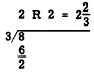The truth of this can be verified another way: If 1 equals 3/3 then 2 equals 6/3 Thus,These examples lead to the following conclusion, which is stated as a rule: To change an improper fraction to a mixed number, divide the numerator by the denominator and write the fractional part of the quotient in lowest terms. Practice problems. Change the following fractions to mixed numbers: 1. 31/20 2. 33/9 3. 65/20 4. 45/8 Answers :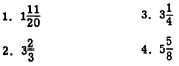OPERATING WITH MIXED NUMBERS In computation, mixed numbers are often unwieldy. As it is possible to change any improper fraction to a mixed number, it is like- wise possible to change any mixed number to an improper fraction. The problem can be reduced to the finding of an equivalent fraction and a simple addition. EXAMPLE: Change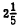to an improper fraction. SOLUTION: Step 1: Writeas a whole number plus a fraction, 2 +1/5. Step 2: Change 2 to an equivalent fraction with a denominator of 5, as follows: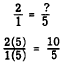Step 3: AddThus,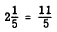EXAMPLE: Writeas an improper fraction. SOLUTION: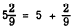Thus,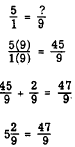In each of these examples, notice that the multiplier used in step 2 is the same number as the denominator of the fractional part of the original mixed number. This leads to the following conclusion, which is stated as a rule: To change a mixed number to an improper fraction, multiply the whole-number part by the denominator of the fractional part and add the numerator to this product. The result is the numerator of the improper fraction; its denominator is the same as the denominator of the fractional part of the original mixed number. Practice problems. Change the following mixed numbers to improper fractions: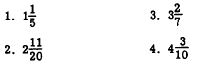Answers: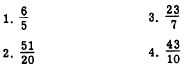NEGATIVE FRACTIONS A fraction preceded by a minus sign is negative. Any negative fraction is equivalent to a positive fraction multiplied by -1. For example,The number -2/5 is read "minus two-fifths." We know that the quotient of two numbers with unlike signs is negative. Therefore,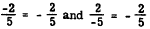This indicates that a negative fraction is equivalent to a fraction with either a negative numerator or a negative denominator. The fraction 2/-5 is read "two over minus five." The fraction 3 is read "minus two over five." A minus sign in a fraction can be moved about at will. It can be placed before the numerator, before the denominator, or before the fraction itself. Thus,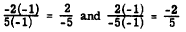Moving the minus sign from numerator to denominator, or vice versa, is equivalent to multiplying the terms of the fraction by -1. This is shown in the following examples: A fraction may be regarded as having three signs associated with it-the sign of the numerator, the sign of the denominator, and the sign preceding the fraction. Any two of these signs may be changed without changing the value of the fraction. Thus,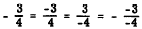OPERATIONS WITH FRACTIONS It will be recalled from the discussion of denominate numbers that numbers must be of the same denomination to be added. We can add pounds to pounds, pints to pints, but not ounces to pints. If we think of fractions loosely as denominate numbers, it will be seen that the rule of likeness applies also to fractions. We can add eighths to eighths, fourths to fourths, but not eighths to fourths. To add 1/5 inch to 2/5 inch we simply add the numerators and retain the denominator unchanged. The denomination is fifths; as with denominate numbers, we add 1 fifth to 2 fifths to get 3 fifths, or 3/5.Integrated Publishing, Inc. - A (SDVOSB) Service Disabled Veteran Owned Small Business